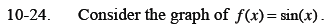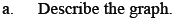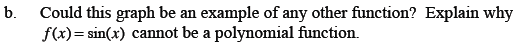Home > CCA2 > Chapter 10 > Lesson 10.1.1 > Problem10-24

10-24.
1. Consider the graph of f(x) = sin(x). 10-24 HW eTool (Desmos). Homework Help ✎

1. Describe the graph.

2. Could this graph be an example of any other function? Explain why f(x) = sin(x) cannot be a polynomial function.What does the graph of y = cos(x) look like?

Polynomial functions are of the form P(x) = axn + bxn − 1 + ... + fx1 + gx0.
How many roots can a polyhomial function have?
How many roots does the sine function have?

Use the eTool below to examine the graph and to create new graphs.
Click the link at right for the full version of the eTool: CCA2 10-24 HW eTool.